# On positive periodic solutions of second-order difference equations with attractive-repulsive singularities

## Abstract

In this paper, sufficient conditions for the existence of positive periodic solutions of the second-order difference equation

$Δ 2 u(t−1)= g ( t ) u μ ( t ) − h ( t ) u λ ( t ) +f(t),t∈Z,$

are established, where $g,h:Z→[0,∞)$ and $f:Z→R$ are T-periodic functions, $λ,μ>0$.

MSC:34B15.

## 1 Introduction

The theory of nonlinear difference equations has been widely used to study discrete models appearing in many fields such as computer science, economics, neural network, ecology, and cybernetics; see, for example, . In recent years, there have been many papers to study the existence of positive periodic solutions for second-order difference equations. By using various methods and techniques, for example, fixed point theorems, the method of upper and lower solutions, coincidence degree theory, and critical point theory, a series of existence results of periodic solutions have been obtained; we refer the reader to  and references therein. However, there are few techniques for studying the existence of positive solutions of difference equations with singularity, and thus, the results in the field are very rare; see . At the same time, we also find that difference equations are closely related to differential equations in the sense that (i) a differential equation model is usually derived from a difference equation, and (ii) numerical solutions of a differential equation have to be obtained by discretizing the differential equation (thus resulting in difference equations). Therefore, it is worthwhile to explore this topic.

Let denote the integer set for $a,b∈Z$ with $a, $[ a , b ] Z :={a,a+1,…,b}$.

In this paper, we are concerned with the existence of positive periodic solutions of the second-order difference equation

$Δ 2 u(t−1)= g ( t ) u μ ( t ) − h ( t ) u λ ( t ) +f(t),t∈Z,$
(1.1)

where $g,h:Z→[0,∞)$ and $f:Z→R$ are T-periodic functions, $λ,μ>0$. By a solution to (1.1) we understand a function $u∈E:={u:Z→R|u(t)=u(t+T)}$ satisfying (1.1). Special cases of Eq. (1.1) are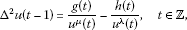(1.2)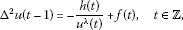(1.3)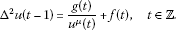(1.4)

In the related literature, it is said that (1.3) has an attractive singularity, whereas (1.4) has a repulsive singularity. The interest in this type of equations began with the paper of Lazer and Solimini , in which the authors provide necessary and sufficient conditions for the existence of periodic solutions of the equation

$u ″ (t)=± h ( t ) u λ ( t ) +f(t),t∈R,$

here $h∈C(R,[0,∞))$ and $f∈C(R,R)$ are T-periodic functions, $λ>0$. Equation (1.2) is interesting due to a mixed type of singularity on the right-hand side. Since the functions g and h are possibly zero on some sets of positive measure, the singularity may combine attractive and repulsive effects. If h, g are positive constants, the singular term can be regarded as a generalized Lennard-Jones force or van der Waals attraction/repulsion force and it is widely used in molecular dynamics to model the interaction between atomic particles (see, for instance, [14, 15] and the references therein). In a different physical context, a periodic solution of Eq. (1.3) can describe a discrete nonlinear Schrödinger equation with a cubic term, then the method of moments leads to the study of a particular case of (1.3); see  for more details. Finally, a third different range of applicability is the evolution of optical pulses in dispersion-managed fiber communication devices .

In spite of the variety of physical applications, the analysis of differential and difference equations with mixed singularities is at this moment very incomplete, and few references can be cited (see [12, 1820]) if compared with the large number of references devoted to singular equations either of attractive or repulsive type (see the review  and the references therein). Our main purpose in this paper is to contribute to the literature trying to fill partially this gap in the study of singularities of mixed type with an approach that should be useful as a starting point for further studies.

The structure of the paper is as follows. Section 2 contains the tools needed in the proofs. In Section 3, we state and prove the main results and develop some corollaries for the equation with a singularity of mixed type. To illustrate the results, an application to the discrete dynamics of a trapless Bose-Einstein condensate is given. This model and related ones deserve a different treatment more oriented to a physical audience, which will be performed elsewhere.

For the sake of brevity, we will use the following notation throughout the paper:

$G= ∑ s = 1 T g(s),H= ∑ s = 1 T h(s),F= ∑ s = 1 T f(s), F + = ∑ s = 1 T [ f ( s ) ] + , F − = ∑ s = 1 T [ f ( s ) ] − .$

## 2 Auxiliary results

Let

$E:= { u : Z → R | u ( t ) = u ( t + T ) }$

under the norm $∥u∥= max t ∈ [ 1 , T ] Z |u(t)|$. Then $(E,∥⋅∥)$ is a Banach space.

The proofs of our results rely on the method of upper and lower functions. The following lemma is classical and can be found, e.g., in . We introduce them in a form suitable for us.

Lemma 2.1 Let there exist positive functions $α,β∈E$ such that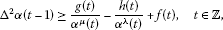(2.1)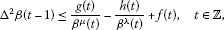(2.2)

and $α(t)≤β(t)$ for $t∈ [ 1 , T ] Z$. Then there exists at least one positive solution to (1.1).

To finish this section, we show a technical bound on the amplitude of oscillation of a periodic function.

Lemma 2.2 Given $v∈E$, then

$M v − m v ≤ T 4 ∑ s = 1 T [ Δ 2 v ( s − 1 ) ] + ,$
(2.3)

where

$M v =max { v ( t ) : t ∈ [ 1 , T ] Z } , m v =min { v ( t ) : t ∈ [ 1 , T ] Z } .$
(2.4)

Moreover, (2.3) is fulfilled as an equality if and only if v is a constant function.

Proof If v is a constant function, then (2.3) follows trivially.

Let v be a non-constant function and choose $t 0 , t 1 ∈ [ 1 , T ] Z$ such that

$v( t 0 )= M v ,v( t 1 )= m v .$

Without loss of generality, we can assume that $t 0 < t 1$. Indeed, in the case where $t 1 < t 0$, we can consider a function −v instead of v, and using the fact that $v∈E$, we have

$∑ s = 1 T [ Δ 2 v ( s − 1 ) ] − = ∑ s = 1 T [ − Δ 2 v ( s − 1 ) ] + .$

Put

$M 1 =max { Δ v ( t ) : t ∈ [ 0 , T ] Z } , m 1 =min { Δ v ( t ) : t ∈ [ 0 , T ] Z } .$
(2.5)

Then, obviously, $M 1 >0$, $m 1 <0$ and by the periodicity of v and $Δv(t)=v(t+1)−v(t)$, we have

$M v − m v = ∑ s = 1 t 0 − 1 Δv(s)+ ∑ s = t 1 T Δv(s)< M 1 (T+ t 0 − t 1 )$
(2.6)

and

$M v − m v =− ∑ s = t 0 t 1 − 1 Δv(s)<− m 1 ( t 1 − t 0 ).$
(2.7)

On the other hand, we have $M v − m v >0$, and thus multiplying of the corresponding sides of (2.6) and (2.7) results in

$( M v − m v ) 2 <− m 1 M 1 (T+ t 0 − t 1 )( t 1 − t 0 ).$
(2.8)

Now, using the inequality $AB≤ 1 4 ( A + B ) 2$, from (2.8) we get

$( M v − m v ) 2 < ( M 1 − m 1 ) 2 T 2 16 ,$

whence the inequality

$M v − m v < T 4 ( M 1 − m 1 )$
(2.9)

follows.

On the other hand, choose $t 2 , t 3 ∈ [ 0 , T ] Z$ such that

$Δv( t 2 )= M 1 ,Δv( t 3 )= m 1 .$

If $t 2 < t 3$, then by using again that v is T-periodic, we have

$M 1 − m 1 = M 1 − Δ v ( 0 ) + Δ v ( T ) − m 1 = ∑ s = 1 t 2 Δ 2 v ( s − 1 ) + ∑ s = t 3 + 1 T Δ 2 v ( s − 1 ) ≤ ∑ s = 1 T [ Δ 2 v ( s − 1 ) ] + .$

If $t 3 < t 2$, then

$M 1 − m 1 = ∑ s = t 3 + 1 t 2 Δ 2 v(s−1)≤ ∑ s = 1 T [ Δ 2 v ( s − 1 ) ] + .$

Consequently, in both cases $t 2 < t 3$ and $t 3 < t 2$, we have $M 1 − m 1 ≤ ∑ s = 1 T [ Δ 2 v ( s − 1 ) ] +$, which together with (2.9) implies (2.3). □

## 3 The main results

The following theorems are the main results of the paper.

Theorem 3.1 Let $H>0$, $F>0$, functions $w,σ∈E$ be such that the equalities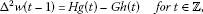(3.1)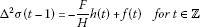(3.2)

are fulfilled, and let there exist $x 0 ∈(0,+∞)$ such that

$x 0 ( w ( t ) − m w ) +σ(t)− m σ ≤ ( H x 0 G H + F ) 1 / λ − ( 1 x 0 H ) 1 / μ ,t∈ [ 1 , T ] Z ,$
(3.3)

where

$m w =min { w ( t ) : t ∈ [ 1 , T ] Z } , m σ =min { σ ( t ) : t ∈ [ 1 , T ] Z } .$
(3.4)

Then the problem (1.1) has at least one positive T-periodic solution.

Proof Put

Obviously, $α∈E$, and in view of (3.1) and (3.2), we have

$Δ 2 α(t−1)= x 0 Hg(t)− ( x 0 G + F H ) h(t)+f(t),t∈Z.$
(3.5)

Moreover, according to (3.3) and (3.4),

(3.6)

Now (3.5) and (3.6) imply

$Δ 2 α(t−1)≥ g ( t ) α μ ( t ) − h ( t ) α λ ( t ) +f(t),t∈Z.$

Consequently, α is a lower function to (1.1).

Further, we can choose $x 1 ∈(0, x 0 )$ such that

$x 1 ( w ( t ) − m w ) +σ(t)− m σ ≤ ( 1 x 1 H ) 1 / μ − ( H x 1 G H + F ) 1 / λ ,t∈ [ 1 , T ] Z ,$
(3.7)

and put

Then $β∈E$, and in view of (3.1) and (3.2), we have

$Δ 2 β(t−1)= x 1 Hg(t)− ( x 1 G + F H ) h(t)+f(t),t∈Z.$
(3.8)

Moreover, according to (3.4) and (3.7),

(3.9)

Now (3.8) and (3.9) imply

$Δ 2 β(t−1)≤ g ( t ) β μ ( t ) − h ( t ) β λ ( t ) +f(t),t∈Z.$
(3.10)

Consequently, β is an upper function to (1.1).

Moreover, (3.6) and (3.9) imply

Thus, the assertion follows from Lemma 2.1. □

Remark 3.1 Note that for every $q∈E$ such that $∑ s = 1 T q(s)=0$, the periodic solution v of the equation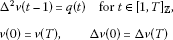is given by the Green formula

$v(t)=c− 1 T [ ∑ s = 1 t − 1 ( T − t ) s q ( s ) + ∑ s = t T t ( T − s ) q ( s ) ] ,$
(3.11)

where $c∈R$. Therefore, the periodic functions w and σ with properties (3.1) and (3.2) exist and, moreover, are unique up to a constant term, the value of which has no influence on the validity of the condition (3.3). A similar observation can be made in relation to the formulations of the theorems given below.

Theorem 3.2 Let $λ>μ$, $H>0$, $G>0$, $F=0$ and functions $w,σ∈E$ be such that the equalities (3.1) and

$Δ 2 σ(t−1)=f(t) for t∈Z$
(3.12)

are fulfilled, and let there exist $x 0 ∈(0,+∞)$ such that

$x 0 ( w ( t ) − m w ) +σ(t)− m σ ≤ ( 1 x 0 G ) 1 / λ − ( 1 x 0 H ) 1 / μ ,t∈ [ 1 , T ] Z ,$
(3.13)

where $m w$ and $m σ$ are defined by (3.4). Then the problem (1.1) has at least one positive T-periodic solution.

Proof Note that the inequality $λ>μ$ implies

$lim x → 0 + ( 1 x H ) 1 / μ − ( 1 x G ) 1 / λ =+∞.$

Therefore, analogously to the proof of Theorem 3.1, one can show that there exist lower and upper functions α, β satisfying $α(t)≤β(t)$, $t∈Z$. Consequently, the assertion follows from Lemma 2.1. □

Corollary 3.1 Let $λ>μ$, $H>0$, $G>0$, and let $w∈E$ be such that (3.1) is fulfilled. Moreover, let

$M w − m w ≤ H 1 + λ λ − μ G 1 + μ λ − μ ( ( 1 + λ ) μ ( 1 + μ ) λ ) ( 1 + λ ) μ λ − μ λ − μ ( 1 + μ ) λ ,$
(3.14)

where $m w$ is given by (3.4) and

$M w =max { w ( t ) : t ∈ [ 1 , T ] Z } .$
(3.15)

Then the problem (1.2) has at least one positive T-periodic solution.

Proof In order to apply Theorem 3.2, put $f≡0$, then $σ≡0$. Take

$x 0 = G μ λ − μ H λ λ − μ ( ( 1 + μ ) λ ( 1 + λ ) μ ) λ μ λ − μ .$

Then (3.14) implies (3.13), and thus the assertion follows from Theorem 3.2. □

Corollary 3.2 Let $λ>μ$, $H>0$ and $G>0$. Moreover, suppose that

$G 1 + λ H 1 + μ ≤ ( 4 T ) λ − μ ( ( 1 + λ ) μ ( 1 + μ ) λ ) ( 1 + λ ) μ ( λ − μ ( 1 + μ ) λ ) λ − μ .$
(3.16)

Then the problem (1.2) has at least one positive T-periodic solution.

Proof By Lemma 2.2, it is easy to verify that

$M w − m w ≤ T 4 GH.$

Now the assertion follows directly from Corollary 3.1. □

To illustrate this latter result, we have selected a concrete physical model studied in . The dynamics of a trapless 3D Bose-Einstein condensate with variable scattering length is ruled by the equation

$Δ 2 u(t−1)= Q 1 u 3 + a ( t ) Q 2 u 4 ,$
(3.17)

where $Q 1$, $Q 2$ are positive parameters and $a(t)$ models the s-wave scattering length, which is assumed to vary T-periodically in time. A negative $a(t)$ corresponds to attractive interactions between the elementary particles. Then the existence of a positive periodic solution of (3.17) is interpreted as a bounded state of the condensate without external trap. Equation (3.17) is a particular case of (1.2) with $μ=3$, $λ=4$. Then a direct consequence of Corollary 3.2 is the existence of a T-periodic solution of (3.17) for any $a∈E$ with $a(t)≤0$, $t∈ [ 1 , T ] Z$, such that

$[ ∑ s = 1 T a ( s ) ] 4 ≥ 4 Q 1 5 T 6 Q 2 4 ( 16 15 ) 15 ≈10.5315 Q 1 5 T 6 Q 2 4 .$

Corollary 3.3 Let $H>0$, $F>0$, $σ∈E$ be such that (3.2) is fulfilled, and let

$( M σ − m σ ) λ F
(3.18)

where $m σ$ is defined by (3.4) and

$M σ =max { σ ( t ) : t ∈ [ 1 , T ] Z } .$

Then the problem (1.3) has at least one positive T-periodic solution.

Proof The assertion follows from Theorem 3.1 with $G=0$. □

Corollary 3.4 Let $H>0$, $F>0$, and let

$( T 4 F + ) λ F≤H.$

Then the problem (1.3) has at least one positive T-periodic solution.

Proof By Lemma 2.2, in view of $F>0$, we have

$M σ − m σ < T 4 F + .$

Now the assertion follows from Corollary 3.3 in a trivial way. □

## References

1. 1.

Kelley WG, Peterson A: Difference Equations, an Introduction with Applications. Academic Press, San Diego; 1991.

2. 2.

Atici FM, Guseinov GS: Positive periodic solutions for nonlinear difference equations with periodic coefficients. J. Math. Anal. Appl. 1999, 232: 166–182. 10.1006/jmaa.1998.6257

3. 3.

Atici FM, Cabada A: Existence and uniqueness results for discrete second-order periodic boundary value problems. Comput. Math. Appl. 2003, 45: 1417–1427. 10.1016/S0898-1221(03)00097-X

4. 4.

Atici FM, Cabada A, Otero-Espinar V: Criteria for existence and nonexistence of positive solutions to a discrete periodic boundary value problem. J. Differ. Equ. Appl. 2003, 9(9):765–775. 10.1080/1023619021000053566

5. 5.

He T, Xu Y: Positive solutions for nonlinear discrete second-order boundary value problems with parameter dependence. J. Math. Anal. Appl. 2011, 379(2):627–636. 10.1016/j.jmaa.2011.01.047

6. 6.

He T, Lu Y, Lei Y, Yang F: Nontrivial periodic solutions for nonlinear second-order difference equations. Discrete Dyn. Nat. Soc. 2011., 2011: Article ID 153082

7. 7.

Ma R, Ma H: Positive solutions for nonlinear discrete periodic boundary value problems. J. Appl. Math. Comput. 2010, 59: 136–141. 10.1016/j.camwa.2009.07.071

8. 8.

Ma R, Lu Y, Chen T: Existence of one-signed solutions of discrete second-order periodic boundary value problems. Abstr. Appl. Anal. 2012., 2012: Article ID 437912

9. 9.

Agarwal RP, O’Regan D: Singular discrete boundary value problems. Appl. Math. Lett. 1999, 12: 127–131.

10. 10.

Agarwal RP, Perera K, O’Regan D: Multiple positive solutions of singular and nonsingular discrete problems via variational methods. Nonlinear Anal. 2004, 58: 69–73. 10.1016/j.na.2003.11.012

11. 11.

Lü H, O’Regan D, Agarwal RP: Positive solution for singular discrete boundary value problem with sign-changing nonlinearities. J. Appl. Math. Stoch. Anal. 2006., 2006: Article ID 46287

12. 12.

Ma R, Lu Y: Positive periodic solutions of second-order difference equations with weak singularities. Adv. Differ. Equ. 2012., 2012: Article ID 90. doi:10.1186/1687–1847–2012–90

13. 13.

Lazer AC, Solimini S: On periodic solutions of nonlinear differential equations with singularities. Proc. Am. Math. Soc. 1987, 99: 109–114. 10.1090/S0002-9939-1987-0866438-7

14. 14.

Johansson K: On separation of phases in one-dimensional gases. Commun. Math. Phys. 1995, 169: 521–561. 10.1007/BF02099311

15. 15.

Rützel S, Lee SI, Raman A: Nonlinear dynamics of atomic-force-microscope probes driven in Lennard-Jones potentials. Proc. R. Soc. Lond., Ser. A, Math. Phys. Eng. Sci. 2003, 459: 1925–1948. 10.1098/rspa.2002.1115

16. 16.

Montesinos GD, Perez-García VM, Torres PJ: Stabilization of solitons of the multidimensional nonlinear Schrödinger equation: matter-wave breathers. Physica D 2004, 191: 193–210. 10.1016/j.physd.2003.12.001

17. 17.

Kunze M: Periodic solutions of a singular Lagrangian system related to dispersion-managed fiber communication devices. Nonlinear Dyn. Syst. Theory 2001, 1: 159–167.

18. 18.

Lei J, Zhang M: Twist property of periodic motion of an atom near a charged wire. Lett. Math. Phys. 2002, 60(1):9–17. 10.1023/A:1015797310039

19. 19.

Torres PJ: Weak singularities may help periodic solutions to exist. J. Differ. Equ. 2007, 232: 277–284. 10.1016/j.jde.2006.08.006

20. 20.

Torres PJ: On periodic solutions of second-order differential equations with attractive-repulsive singularities. J. Differ. Equ. 2010, 248: 111–126. 10.1016/j.jde.2009.07.008

21. 21.

Rachunková I, Rachunek L: Singular discrete second order BVPs with p -Laplacian. J. Differ. Equ. Appl. 2006, 12: 811–819. 10.1080/10236190600734200

22. 22.

Rachunková I, Rachunek L: Singular discrete and continuous mixed boundary value problems. Math. Comput. Model. 2009, 49: 413–422. 10.1016/j.mcm.2008.09.004

23. 23.

Rachunková I, Staněk S, Tvrdý M: Singularities and Laplacians in boundary value problems for nonlinear ordinary differential equations. 3. In Handbook of Differential Equations: Ordinary Differential Equations. Elsevier, Amsterdam; 2006.

## Acknowledgements

The authors are very grateful to the anonymous referees for their valuable suggestions. This work was supported by the NSFC (No. 11061030), NSFC (No. 11126296), the Fundamental Research Funds for the Gansu Universities.

## Author information

Authors

### Corresponding author

Correspondence to Yanqiong Lu.

### Competing interests

The authors declare that they have no competing interests.

### Authors’ contributions

The authors have achieved equal contributions to each part of this paper. All the authors read and approved the final manuscript.

## Rights and permissions

Reprints and Permissions

Lu, Y., Ma, R. On positive periodic solutions of second-order difference equations with attractive-repulsive singularities. Adv Differ Equ 2012, 186 (2012). https://doi.org/10.1186/1687-1847-2012-186